1. /
2. CBSE
3. /
4. Class 09
5. /
6. Science
7. /
8. NCERT Solutions for Class...

# NCERT Solutions for Class 9 Science Atoms and Molecules part 2

NCERT solutions for Science Atoms and Molecules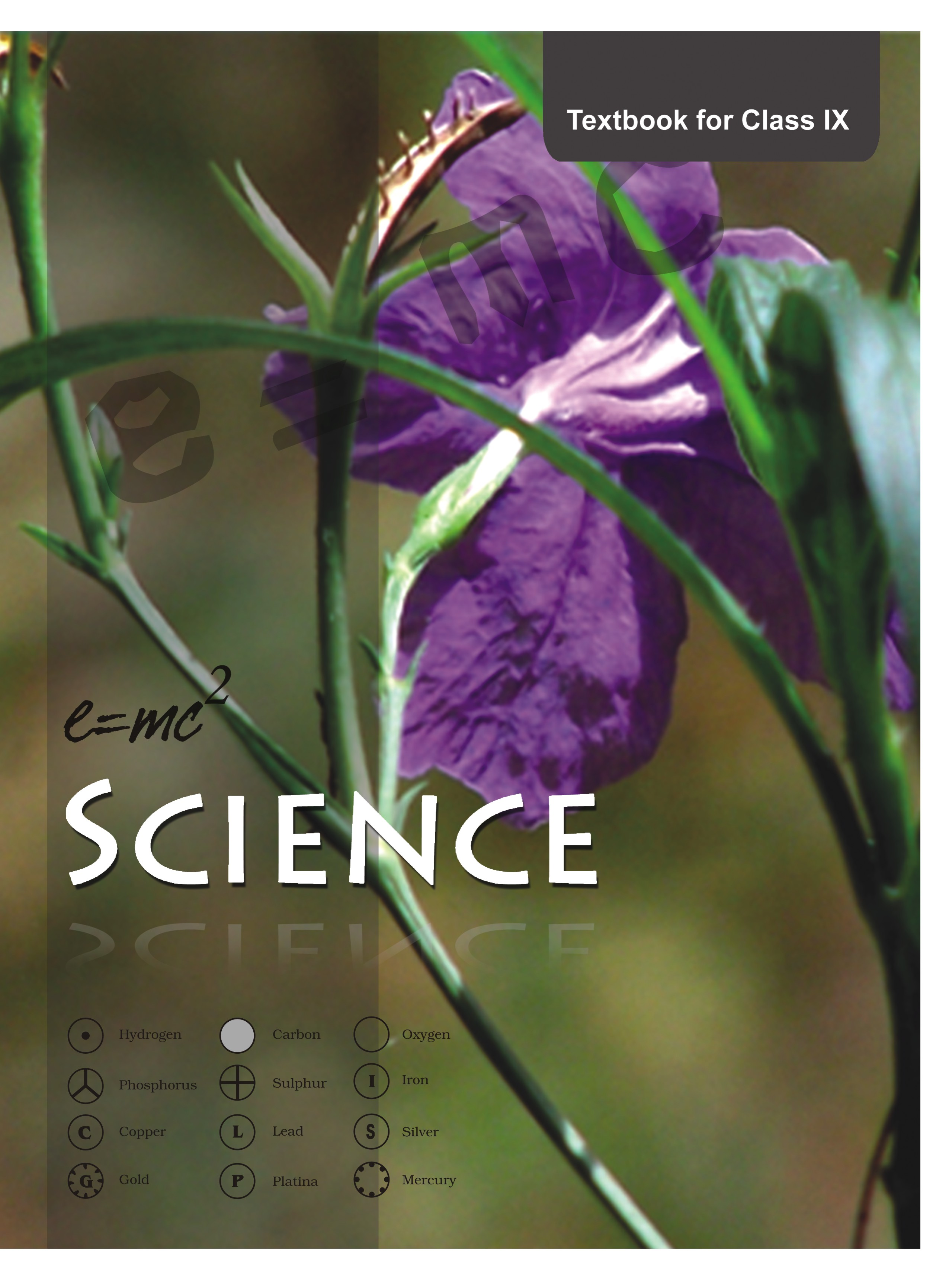## NCERT Class 9 Science Chapter wise Solutions

• 01 –  Matter in Our Surroundings
• 02 – Is Mattter Around us Pure
• 03 – Atoms and Molecules
• 04 – Structure of the Atom
• 05 – The Fundamental Unit of Life
• 06 – Tissues
• 07 – Diversity in Living Organisms
• 08 – Motion
• 09 – Force and Laws of Motion
• 10 – Gravitation
• 11 – Word and Energy
• 12 – Sound
• 13 – Why Do We Fall Ill
• 14 – Natural Resources
• 15 – Improvement in Food Resources

## NCERT Solutions for Class 9 Science Atoms and Molecules part 2

###### 1.A 0.24 g sample of compound of oxygen and boron was found by analysis to contain 0.096 g of boron and 0.144 g of oxygen. Calculate the percentage composition of the compound by weight.

Ans. Mass of the given sample compound = 0.24g

Mass of boron in the given sample compound = 0.096g

Mass of oxygen in the given sample compound = 0.144g

% composition of compound = % of boron and % of oxygen

Therefore % of boron = mass of boron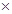100/mass of the sample compound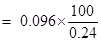= 40%

Therefore % of oxygen = mass of oxygen100/mass of the sample compound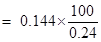= 60%

NCERT Solutions for Class 9 Science Atoms and Molecules part 2

###### 2.When 3.0 g of carbon is burnt in 8.00 g oxygen, 11.00 g of carbon dioxide is produced. What mass of carbon dioxide will be formed when 3.00 g of carbon is burnt in 50.00 g of oxygen? Which law of chemical combination will govern your answer?

Ans. According to the law of chemical combination of constant proportions “in a chemical compound the elementary constituents always combine in constant proportions by weight/mass”. Therefore, whether 3 g carbon is burnt in 8 g oxygen or 3g carbon is burnt in 50g oxygen in both cases only 11g carbon dioxide will be formed.

###### 3.What are polyatomic ions? Give examples.

Ans. When two or more atoms in a group is having a charge, such is called a polyatomic ion. For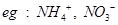etc.

NCERT Solutions for Class 9 Science Atoms and Molecules part 2

###### 4.Write the chemical formulae of the following.

(a) Magnesium chloride

(b) Calcium oxide

(c) Copper nitrate

(d) Aluminium chloride

(e) Calcium carbonate.

Ans.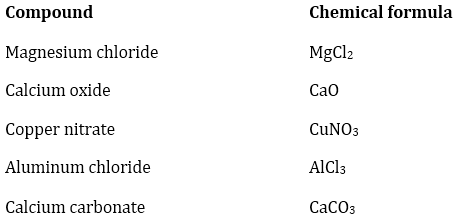###### 5.Give the names of the elements present in the following compounds.

(a) Quick lime

(b) Hydrogen bromide

(c) Baking powder

(d) Potassium sulphate.

Ans.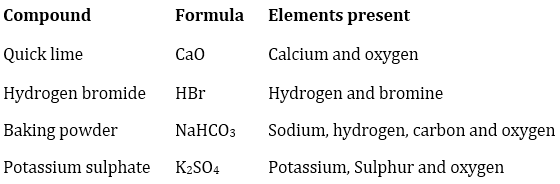NCERT Solutions for Class 9 Science Atoms and Molecules part 2

###### 6. Calculate the molar mass of the following substances.

(a) Ethyne,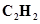(b) Sulphur molecule,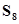(c) Phosphorus molecule,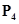(Atomic mass of phosphorus= 31)

(d) Hydrochloric acid, HCl

(e) Nitric acid,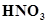Ans. (a)Ethyene =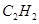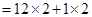=24 + 2= 26 u =26 g

(b)Sulphur molecular =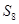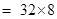= 256 u= 256 g

(c)Phosphorus molecule =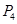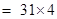= 124 u= 124 g

(d)Hydrochloric acid = HCl = 1+ 35.5 = 36.5 u= 36.5 g

(e)Nitric acid =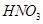= 1 + 14 +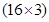= 15 + 48 = 63 u =63 g

NCERT Solutions for Class 9 Science Atoms and Molecules part 2

###### 7.What is the mass of—

(a) 1 mole of nitrogen atoms?

(b) 4 moles of aluminium atoms (Atomic mass of aluminium= 27)?

(c) 10 moles of sodium sulphite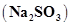?

Ans. (a)Atomic mass of nitrogen is 14 u.

therefore 1 mol of N = 14g

(b)Atomic mass of aluminium = 27u

therefore 1 mol of Al = 27g and so 4 mol of Al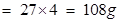(c)molecular mass of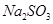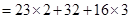= 46 + 32 + 48 = 126 u

therefore 1 mol ofhas weight/mass 126g.

hence, 10 mol of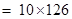= 1260g

###### 8.Convert into mole.

(a) 12 g of oxygen gas

(b) 20 g of water

(c) 22 g of carbon dioxide.

Ans. (a)molecular mass of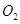= 32 u= 32g(1 mole)

since 32 g of=1mole then 12g of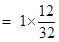=0.375mole.

(b)molecular mass of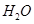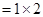+ 16 = 18 u= 18g(1mole)

20g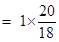= 1.11mole.

(c)molecular mass of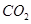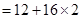= 12 + 32 = 44 u= 44g (1mole)

22g of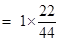= 0.5mole.

NCERT Solutions for Class 9 Science Atoms and Molecules part 2

###### 9.What is the mass of:

(a) 0.2 mole of oxygen atoms?

(b) 0.5 mole of water molecules?

Ans. (a) since 1 mole of O = atomic mass of O = 16u=16g

then 0.2mole of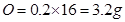(b)1mol of H2O = molecular mass of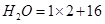=18 u =18g

then 0.5mol of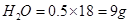###### 10.Calculate the number of molecules of sulphur (S8) present in 16 g of solid sulphur.

Ans. 1mol of= molecular mass of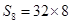= 256u=256g

since 256g of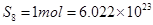atoms (Avogadro number)

16g of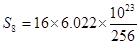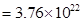molecules

NCERT Solutions for Class 9 Science Atoms and Molecules part 2

###### 11.Calculate the number of aluminium ions present in 0.051 g of aluminium oxide.

(Hint: The mass of an ion is the same as that of an atom of the same element. Atomic mass of Al = 27 u)

Ans. 1mol of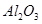= molecular mass of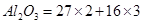= 102u=102g

aluminium ions present in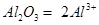102 g ofcontains aluminium ions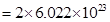then 0.051 gcontains aluminium ions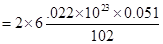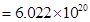## NCERT Solutions for Class 9 Science

NCERT Solutions Class 9 Science PDF (Download) Free from myCBSEguide app and myCBSEguide website. Ncert solution class 9 Science includes text book solutions .NCERT Solutions for CBSE Class 9 Science have total 15 chapters. 9 Science NCERT Solutions in PDF for free Download on our website. Ncert Science class 9 solutions PDF and Science ncert class 9 PDF solutions with latest modifications and as per the latest CBSE syllabus are only available in myCBSEguide.

## CBSE app for Class 9

To download NCERT Solutions for class 9 Science, Computer Science, Home Science,Hindi ,English, Maths Social Science do check myCBSEguide app or website. myCBSEguide provides sample papers with solution, test papers for chapter-wise practice, NCERT solutions, NCERT Exemplar solutions, quick revision notes for ready reference, CBSE guess papers and CBSE important question papers. Sample Paper all are made available through the best app for CBSE students and myCBSEguide website.### Test Generator

Create question paper PDF and online tests with your own name & logo in minutes.### myCBSEguide

Question Bank, Mock Tests, Exam Papers, NCERT Solutions, Sample Papers, Notes

### 1 thought on “NCERT Solutions for Class 9 Science Atoms and Molecules part 2”

1. This is osw…